## Profit and Loss For SBI PO Set – 3

1) Fresh nuts contains 75% of water by weight, where as dry nuts contains 50% of water by weight. Smith buys 50 kg of fresh nuts for a total price of Rs.150. At what price should he sell the dry nuts (fresh nuts are dried up)to earn 20% profit?

a)  5 1/5

b) 8 1/5

c) 9 1/5

d) 7 1/5

e) None of these

a)  5 1/5

Explanation

Shalini share: Nalini share

80000+(80000+20000):80000+(80000-30000)
.180000:130000

18:13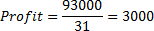Nalini share=3000×13=Rs.39000

2) A shopkeeper sells a transistors at 15% above its cost price. If he had bought it at 5% more than what he paid for it and sold it for Rs.8 more, he would have gained 10%. The cost price of the transistor is?

a) Rs.1600

b) Rs.1500

c) Rs.1400

d) Rs.1300

e) None of these

c) Rs.1400

Explanation

Selling price 94%=5076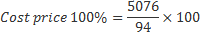=Rs.5400

3)  A seller uses 920 gm in place of one kg to sell his goods. When he sells his article at 15% gain on cost price, the actual percentage of profit is:

a) 25

b) 30

c) 20

d) 15

e) 16

a) 25

Explanation

Let CP = 100 & SP = 115

But he also uses 920 gm in place of 1kg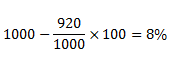Also earn = 8% profit

Now actual CP = 100 – 8 = 92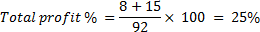4) A bakery bakes cake with expectation that it will earn a profit of 40% by selling each cake at marked price. But during the delivery to showroom 16% of the cakes were completely damaged and hence could not be sold. 24% of the cakes were slightly damaged and hence could be sold at 80% of the cost price. The remaining 60% of the cakes were sold at marked price. What is the percentage profit in the whole consignment?

a)  3.2

b) 2.4

c) 2.8

d) 4.2

e) 3.6

a)  3.2

Explanation

Let total no’s of cake = 100

CP of each cake = 1 Rs. And MP of each coke = 1.4

SP of 24% cake = 24 ×0.8 = 19.2

SP of 60% cake = 60 ×1.4 = 84

Total SP = 84 + 19.2 = 103.2

Profit = 103.2 – 100

Profit = 3.2%

5) A can do a job in 16 days and B can do the same job in 12 days. With help of C, they did the job in 4 days only. Then, C alone can do the job in

a) 10/3 days

b) 11/4 days

c) 48/5 days

d) 71/9 days

e) 57/5 days

c) 48/5 days

Explanation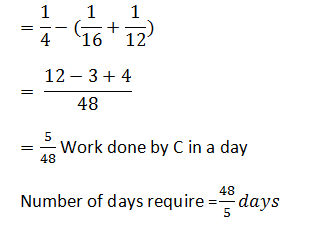6) A shopkeeper gains 10% after allowing a discount of 25% on the market price of an article) Find his profit %, if the articles are sold at market price allowing no discount?

a) 50%

b) 53.75%

c) 47%

d) 40%

e) None of these

c) 47%

Explanation

Let as assume C.P = 100

After adding profit ‘X’ rs, it become 100 + x = S.P

Allowing 25% discount on SP means  75/ 100(100+x) = 100+ 10 = 110

X = 47%

7) Two successive discounts of a% and b% on the marked price of an article are equivalent to the single discount of

a) (a + b)%

b) (a + b –((ab))/100)%

c) (a + /100%)

d)  (a+b)/2%

e) None of these

b) (a + b –((ab))/100)%

Explanation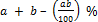8) A Women buys Rice for Rs.4800. She sells one-third of it at a profit of 10%. At what per cent  gain should she sell the remaining two-third so as to make an overall profit of 20% on the whole transaction?

a) 25%

b) 20%

c) 35%

d) 30%

e) None of these

a) 25%

Explanation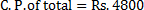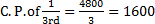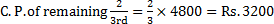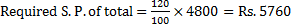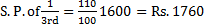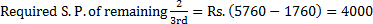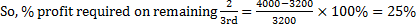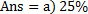9) Pankaj purchased an item for Rs. 7,500 and sold it at the gain of 24%. From that amount he purchased another item and sold it at the loss of 20%. What is his overall gain/loss?

a) Loss of Rs. 140

b) Gain of Rs. 60

c) Loss of Rs. 40

d) Neither gain nor loss

e) None of these

e) None of these

Explanation

Cost price 1=7500

Profit (24%), selling price 124%=124%7500=9300

Now, cost price 2=9300

Loss 20%, Selling price=80%9300=7440

compare two cost prices=7500-7440

Loss =Rs.60

10) Rita purchased an item of Rs. 6500 and sold it at the loss of 20%. From that amount she purchased another item and sold it at the profit of 25%. What is her overall gain/loss?

a) Loss of Rs. 500

b) Gain of Rs. 500

c) Gain of Rs. 250

d) Neither gain nor loss

e) None of these

d) Neither gain nor loss

Explanation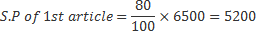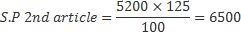Clearly there is no profit, no loss.